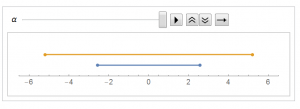8
Oct 16

## The pearls of AP Statistics 32

Student's t distribution: one-line explanation of its origin

They sayWe’ll now learn about a confidence interval that applies even for small sample sizes… Suppose we knew the standard deviation,$\sigma/\sqrt{n}$, of the sample mean. Then, with the additional assumption that the population is normal, with small$n$ we could use the formula$\bar{x}\pm z\sigma/\sqrt{n}$, for instance with$z = 1.96$ for 95% confidence. In practice, we don’t know the population standard deviation σ. Substituting the sample standard deviation s for σ to get$se=s/\sqrt{n}$ then introduces extra error. This error can be sizeable when$n$ is small. To account for this increased error, we must replace the z-score by a slightly larger score, called a t-score. The confidence interval is then a bit wider. (Agresti and Franklin, p.369)

I say: The opening statement in italic (We’ll now learn about...) creates the wrong impression that the task at hand is to address small sample sizes. The next part in italic (To account for this increased error...) confuses the reader further by implying that

1) using the sample standard deviation instead of the population standard deviation and

2) replacing the z score by the t score

are two separate acts. They are not: see equation (3) below. The last proposition in italic (The confidence interval is then a bit wider) is true. It confused me to the extent that I made a wrong remark in the first version of this post, see Remark 4 below.

### Preliminaries

William Gosset published his result under a pseudonym ("Student"), and that result was modified by Ronald Fisher to what we know now as Student's t distribution. Gosset with his statistic wanted to address small sample sizes. The modern explanation is different: the t statistic arises from replacing the unknown population variance by its estimator, the sample variance, and it works regardless of the sample size. If we take a couple of facts on trust, the explanation will be just a one-line formula.

Let$X_1,...,X_n$ be a sample of independent observations from a normal population.

Fact 1. The z-score of the sample mean

(1)$z_0=\frac{\bar{X}-E\bar{X}}{\sigma(\bar{X})}=\frac{\bar{X}-\mu}{\sigma/\sqrt{n}}$

is a standard normal variable.

Fact 2. The sample variance$s^2=\frac{1}{n-1}\sum(X_i-\bar{X})^2$ upon scaling becomes a chi-square variable. More precisely, the variable

(2)$\chi^2_{n-1}=\frac{(n-1)s^2}{\sigma^2}$

is a chi-square with$n-1$ degrees of freedom.

Fact 3. The variables in (1) and (2) are independent.

### Intuitive introduction to t distribution

When a population parameter is unknown, replace it by its estimator. Following this general statistical idea, in the situation when$\sigma$ is unknown, instead of (1) consider

(3)$t=\frac{\bar{X}-\mu}{s/\sqrt{n}}$ (dividing and multiplying by$\sigma$$=\frac{\bar{X}-\mu}{\sigma/\sqrt{n}}\frac{1}{\sqrt{s^2/\sigma^2}}$ (using (1), (2))$=\frac{z_0}{\sqrt{\chi^2_{n-1}/(n-1)}}.$

By definition and because the numerator and denominator are independent, the last expression is a t distribution with$n-1$ degrees of freedom. This is all there is to it.

### Concluding remarks

Remark 1. When I give the definitions of chi-square, t statistic and F statistic, my students are often surprised. This is because there is no reference to samples. To be precise, it is better to remember that the way I define them, they are random variables and not statistics and using "distribution" or "variable" would be more appropriate than "statistic". A statistic, by definition, is a function of observations. The variable we start with in (3) is, obviously, a statistic. Equation (3) means that that statistic is distributed as t with n-1 degrees of freedom.

Remark 2. Many AP Stats books claim that a sum of normal variables is normal. In fact, for this to be true we need independence of the summands. Under our assumption of independent observations and normality, the sum$X_1+...+X_n$ is normal. The variable in (1) is normal as a linear transformation of this sum. Since its mean is zero and its variance is 1, it is a standard normal. We have proved Fact 1. The proofs of Facts 2 and 3 are much more complex.

Remark 3. The t statistic is not used for large samples not because it does not work for large n but because for large n it is close to the z score.Remark 4. Taking the t distribution defined here as a standard t, we can define a general t as its linear transformation,$GeneralT=\sigma*StandardT+\mu$ (similarly to general normals). Since the standard deviation of the standard t is not 1, the standard deviation of the general t we have defined will not be$\sigma$. The general t is necessary to use the Mathematica function StudentTCI (confidence interval for Student's t). The t score that arises in estimation is the standard t. In this case, confidence intervals based on t are indeed wider than those based on z. I apologize for my previous wrong comment and am posting this video. See an updated Mathematica file.